## Geometry Teacher’s Guide by AskDrCallahan – PDF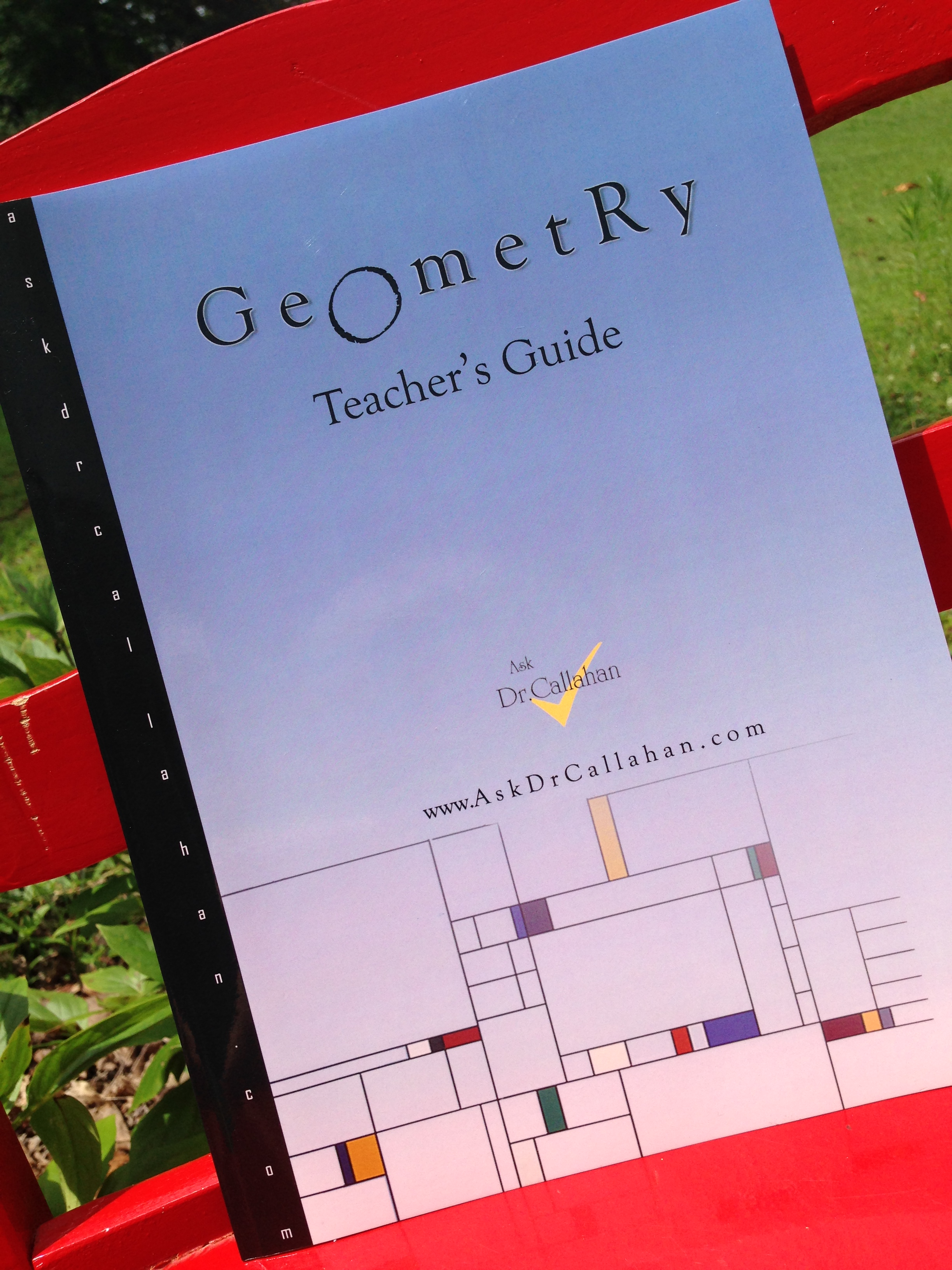This teacher’s guide contains a different syllabus from the Teacher’s Guide written by Jacobs. This guide has carefully selected problems assigned by Dr. Callahan rather than working all the problems, as well as, video viewing assignments.

Posted on

## Jacobs Geometry Chapter 15 Lesson 1 Problem 57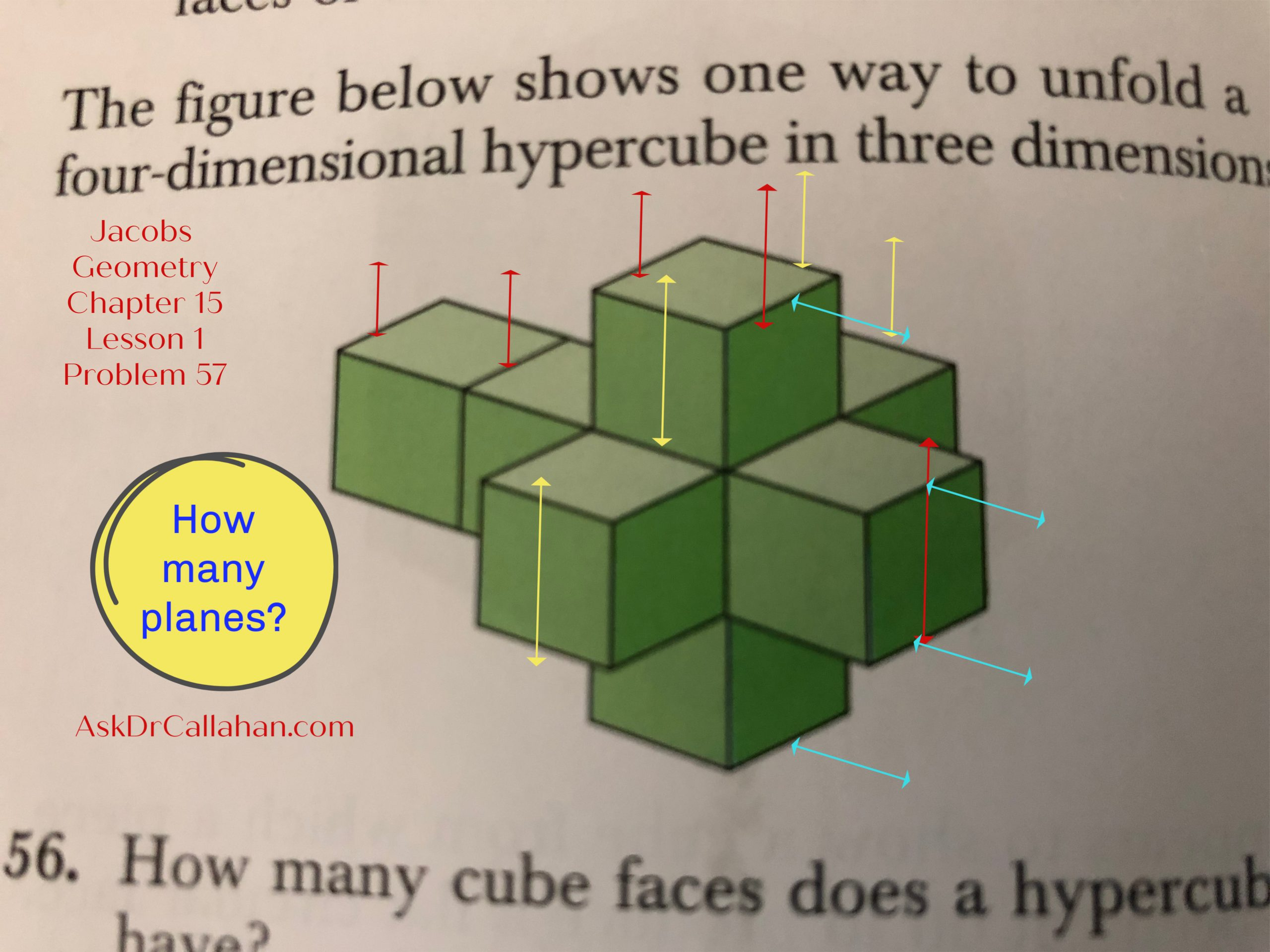In Jacobs Geometry Chapter 15 Lesson 1 problem 57, we are asked,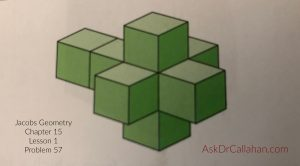“In how many planes do the square faces of the cube faces of the unfolded hypercube lie?”

The Solutions Manual says 13 planes. Let’s see how they got that number.

Imagine the red, yellow, and blue lines drawn on the hypercube as planes.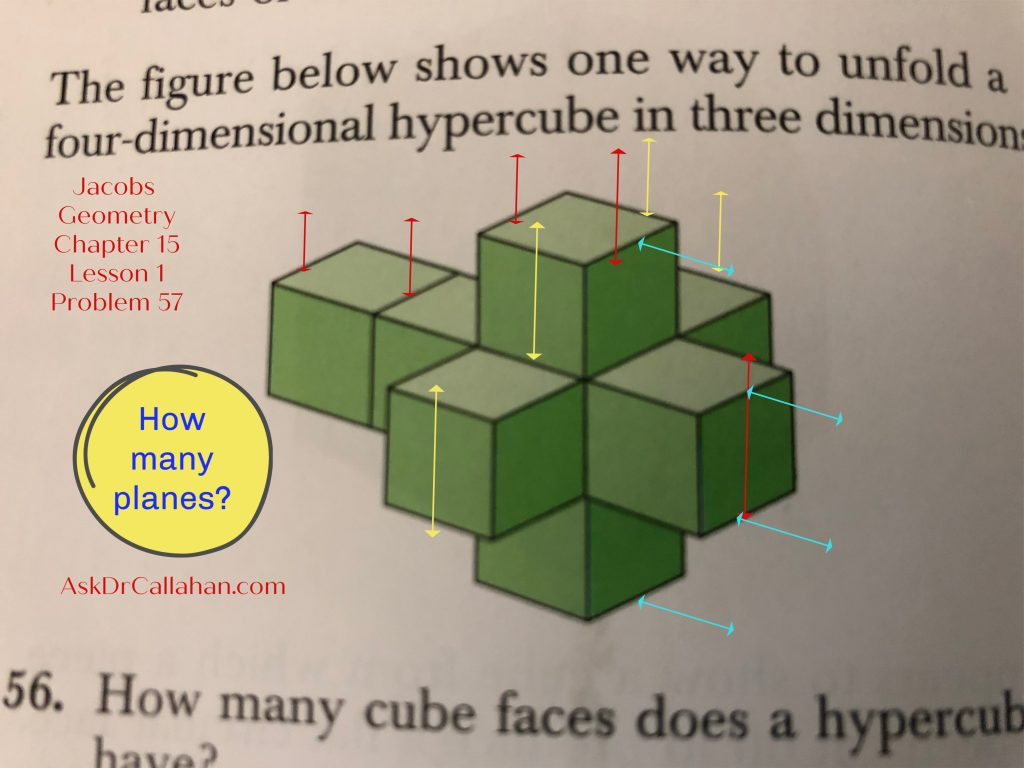Counting vertical planes from right to left: marked in red we have 5.

Counting vertical planes from front to back: marked in yellow we have 4.

Counting horizontal planes from bottom to top: marked in blue we have 4.

5 + 4 + 4 = 13 planes.

Need help with your homework problem? AskDrCallahan video instruction students can submit questions to support@askdrcallahan.com or through our website form on the student help page. Please always include the course, chapter, lesson, and problem number. Any description of your struggle is also very helpful so we get directly to your issue.  We love to help.

Posted on

## Geometry: Note on Missing Chapters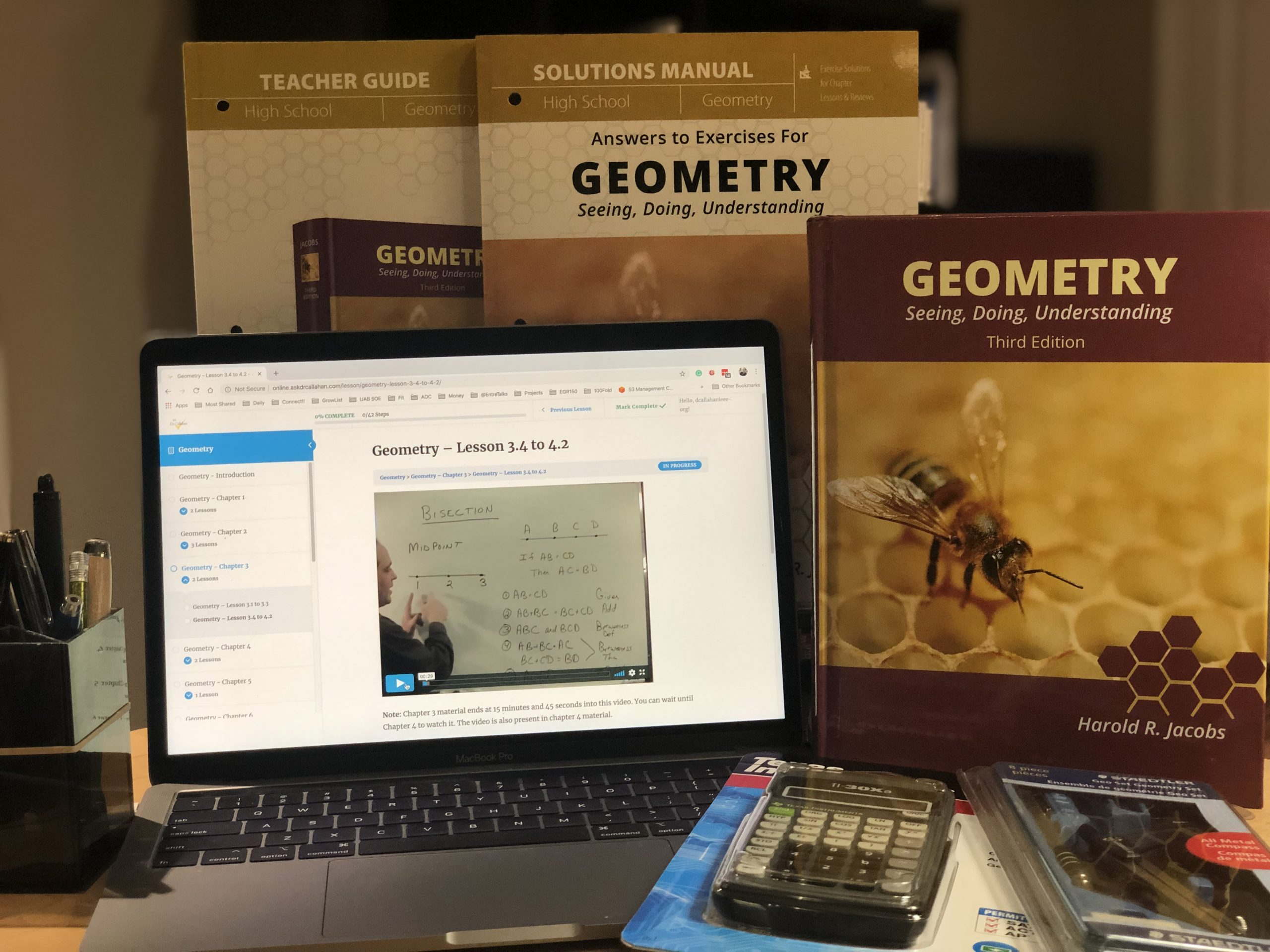## Geometry Course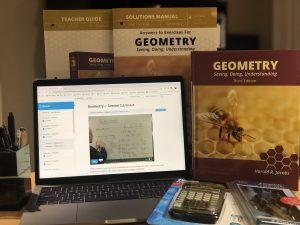Chapters 13 and 16 were originally omitted from our Geometry course for time constraints. The concepts in those chapters are advanced, though not difficult. Students will not likely see them on the ACT/SAT or even in college, except for specialized fields. When needed in a college track, they will be covered anew. They are often skipped at the high school level.

## Geometry Chapter 13 and Chapter 16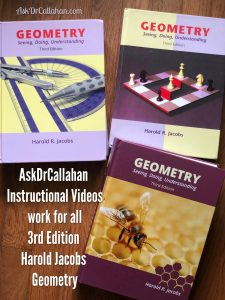However, we now recommend Chapter 13 be covered. Chapter 14 briefly refers to concepts introduced there and some students need the information. Others will be okay just referring back. Most of our students still skip chapter 13. We still consider Chapter 16 optional for everyone.
We have lectures for both chapters now on our website for FREE.
Remember that our video instruction, online or DVD, can be used with any printing of the 3rd Edition of Jacobs Geometry.
Posted on

## Geometry Ch11 test #7b

Question from Anya
Course: Geometry
Chapter/Lesson/Problem11 test7b
Issue: I’m supposed to find Angle APM. To find the angle, I used tan APM=20/70, which gives 15.9 degrees. The solutions manual used sin APM=20/70, which gives 16.6 degrees. Sine is opposite/hypotenuse. However, it seems to me that the given measurements of the problem are opposite side (20) to the adjacent side (70). Which is correct? Thank you!
Yes, you are correct. That should be tangent as you stated.
tan(APM) = 20/70
Posted on

## Geometry 14.2 #22-23

Question from Joy:

We are in Geometry 3 rd edition. Chapter 14 lesson 2 number 22-23. Page 582.
How do you get 108?

The answer in key says 3 x 180/5?  Why? I think I missed something important yet simple. I was thinking divide 360/5. What an I doing wrong?  Thanks!

I have to admit – I could not find this in the book. I think it SHOULD be there – but could not find it.

The answer is the a triagle has interior angles that add up to 180 (we know that – right)

So each time we add a side (triangle to square) we add 180.

So in #22, he is saying we have 180 3 times (triangle plus 2 sides adding 2 more 180s) as the total angles and then dived by the number of sides to get 108.

Again – I do not see this in the book.

Hope this helps

dwc

Posted on

## Geometry Ch13.6 #32

For Chap 13, Lesson 6, #32, the basis for the answers is the figure for #6.  If we look carefully at the grid, applying the Pythagorean Theorem gives

XY^2 = 2^2 + 2^2 = 8,

so XY = sqrt8 = 2sqrt2.

YZ^2 = 1^2 + 1^2 = 2,

so YZ =sqrt2.

Therefore XY = 2YZ.
Harold Jacobs

—

—

Posted on

## The Different Geometry Textbooks and Videos

The 3rd edition of the Harold Jacobs Geometry textbook has gone through three different printings with 3 different covers and ISBNs. If you are picking a text and looking at used options, it can be confusing. So let me try to help.

First, all 3 versions are basically the same – as long as they say 3rd edition. They look like the photos below.

### The Three Geometry Textbook Versions that Still Work

The original published by Freeman – ISBN: 978-0-7167-4361-3 and the corresponding Teachers Guide which contains the solutions to the problems. This book had a separate Test Bank for tests. The second printing published by My Father’s World – ISBN: 978-1-61999-109-5 and the corresponding Teachers Guide which contained the tests and the Answer Key.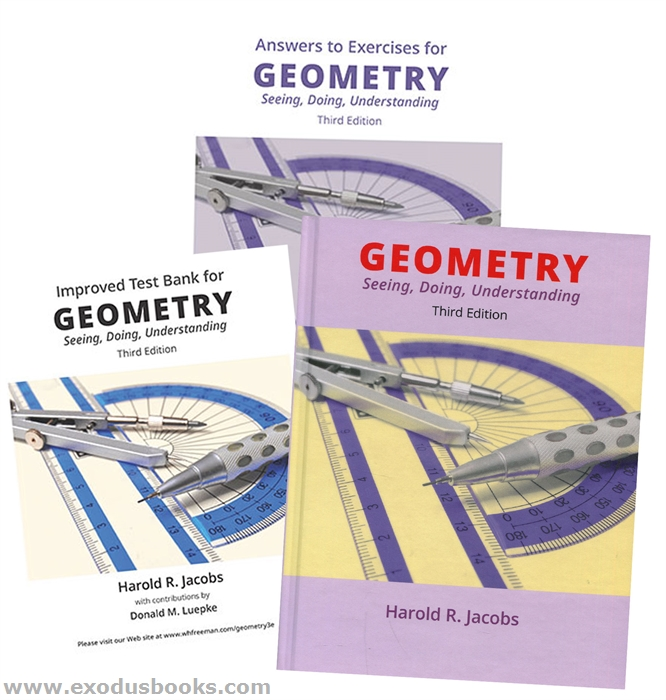The third printing published by Master Books – ISBN: 9787-1-68344-020-8. This is the latest and if you are buying new, this is what you are getting. The tests are in the Teacher’s Guide and the answers are in the Solutions Manual. You will need all 3 books for the course.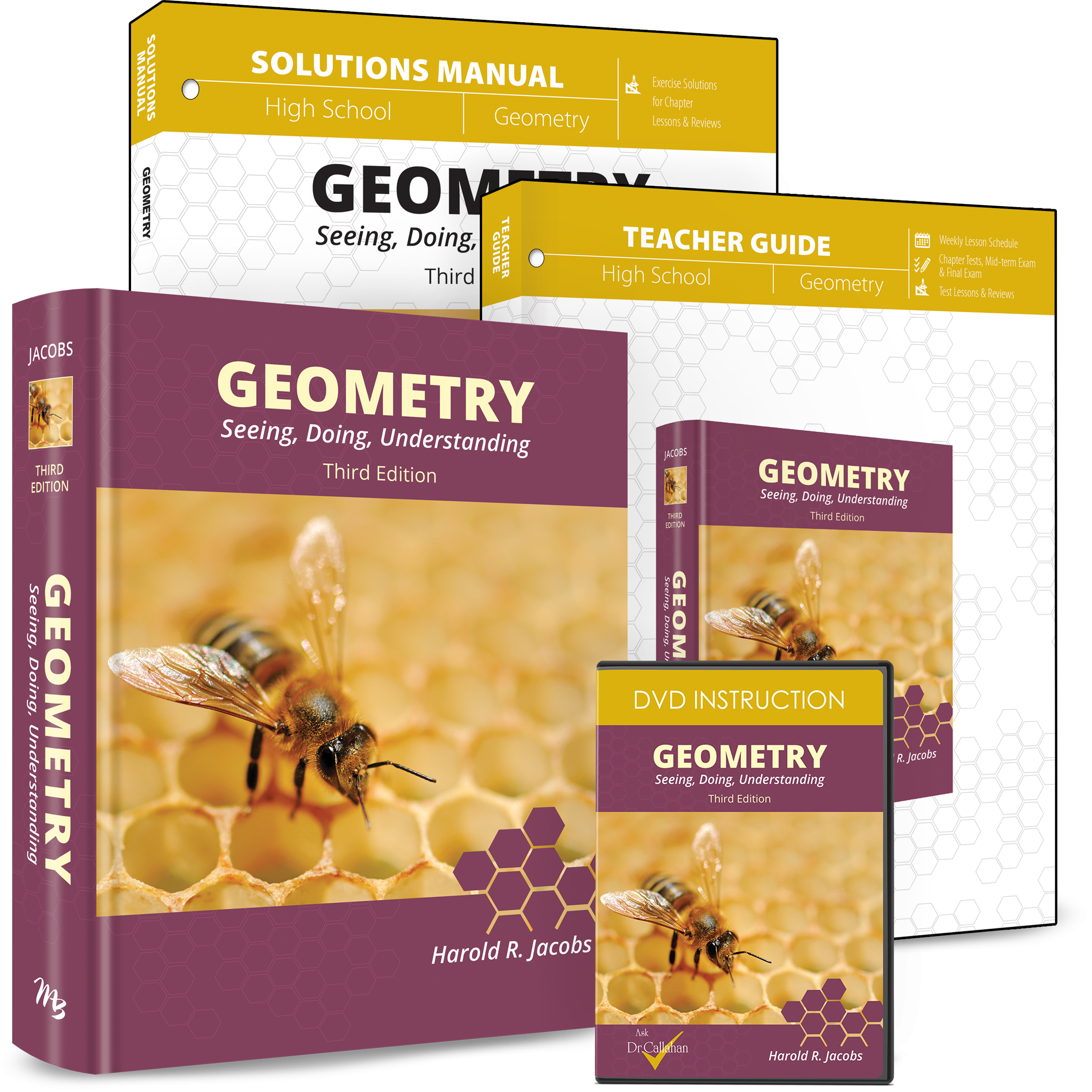### The Geometry Videos that Support the above Books

All 3 of the printings will work with the AskDrCallahan videos. The various printings have minor changes in page numbers, drawings, and some problems have changed – but the basic content remains the same. But, just to be more confusing, the videos come in 3 different packages. All are the same content but they might look different. All require the student to have one of the 3 textbooks, the tests, and a set of solutions.

1 – The online videos, offered by AskDrCallahan, contain the same instruction, and bypass the need for DVDs and a DVD player. They are the same content as on the DVD. The online videos come in two options.

• Monthly option – Pay for the course monthly and cancel anytime by logging into your AskDrCallahan account (or email us to cancel). This option is perfect if you think you will need just a few months.
• Lifetime access – If you need a full year, or have siblings who might use the course later, then this is your option. One price one time.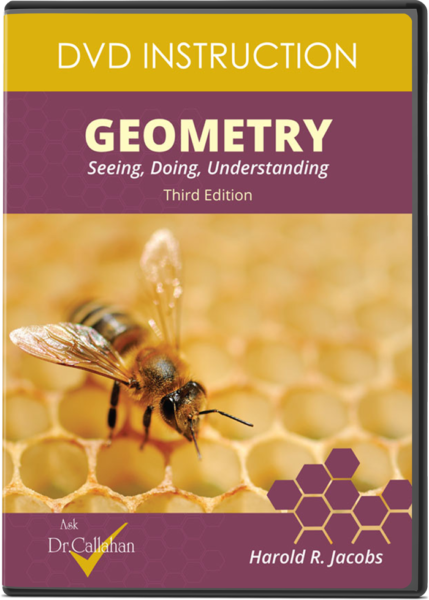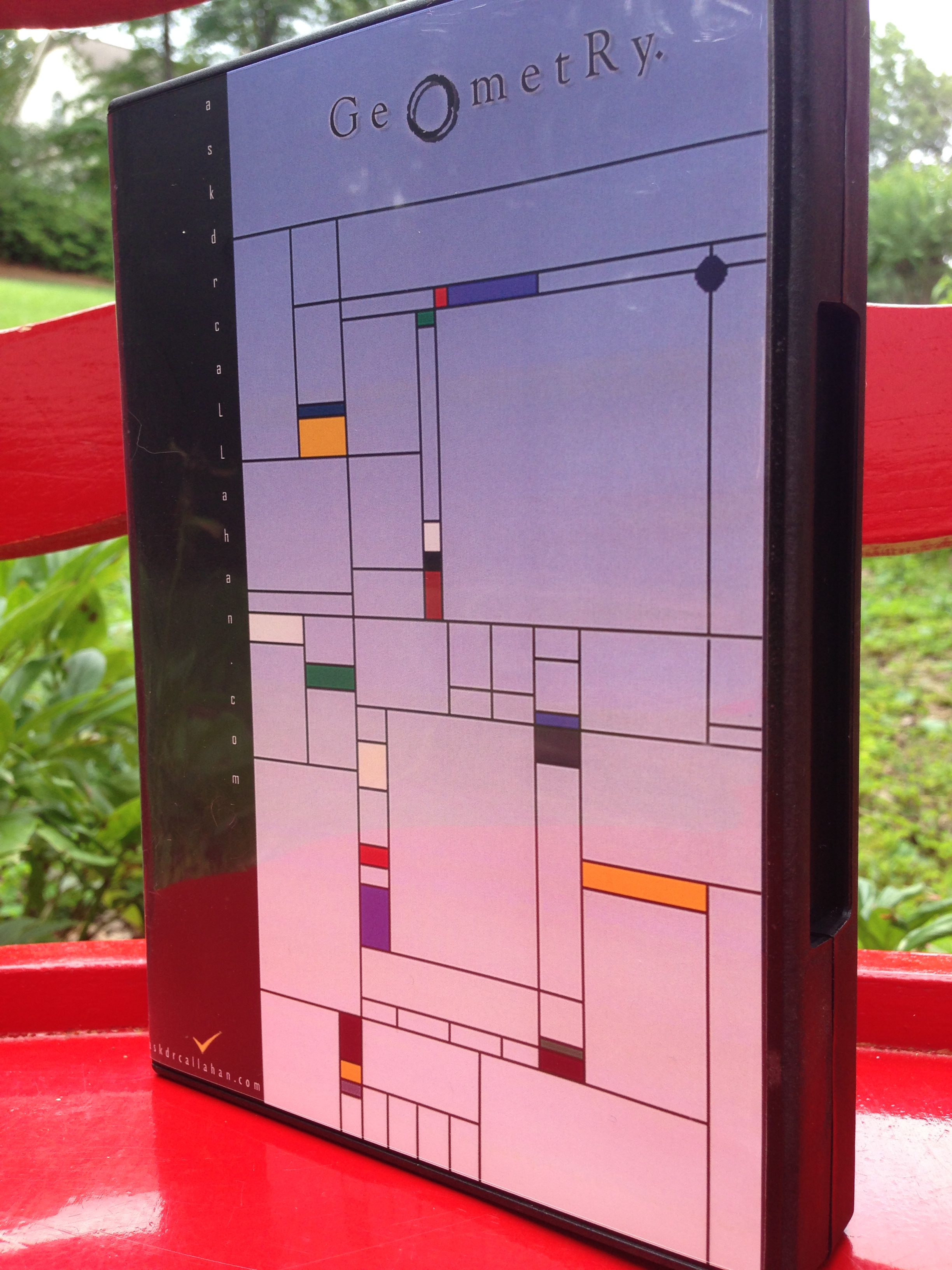Posted on

## Geometry Ch8 Review #32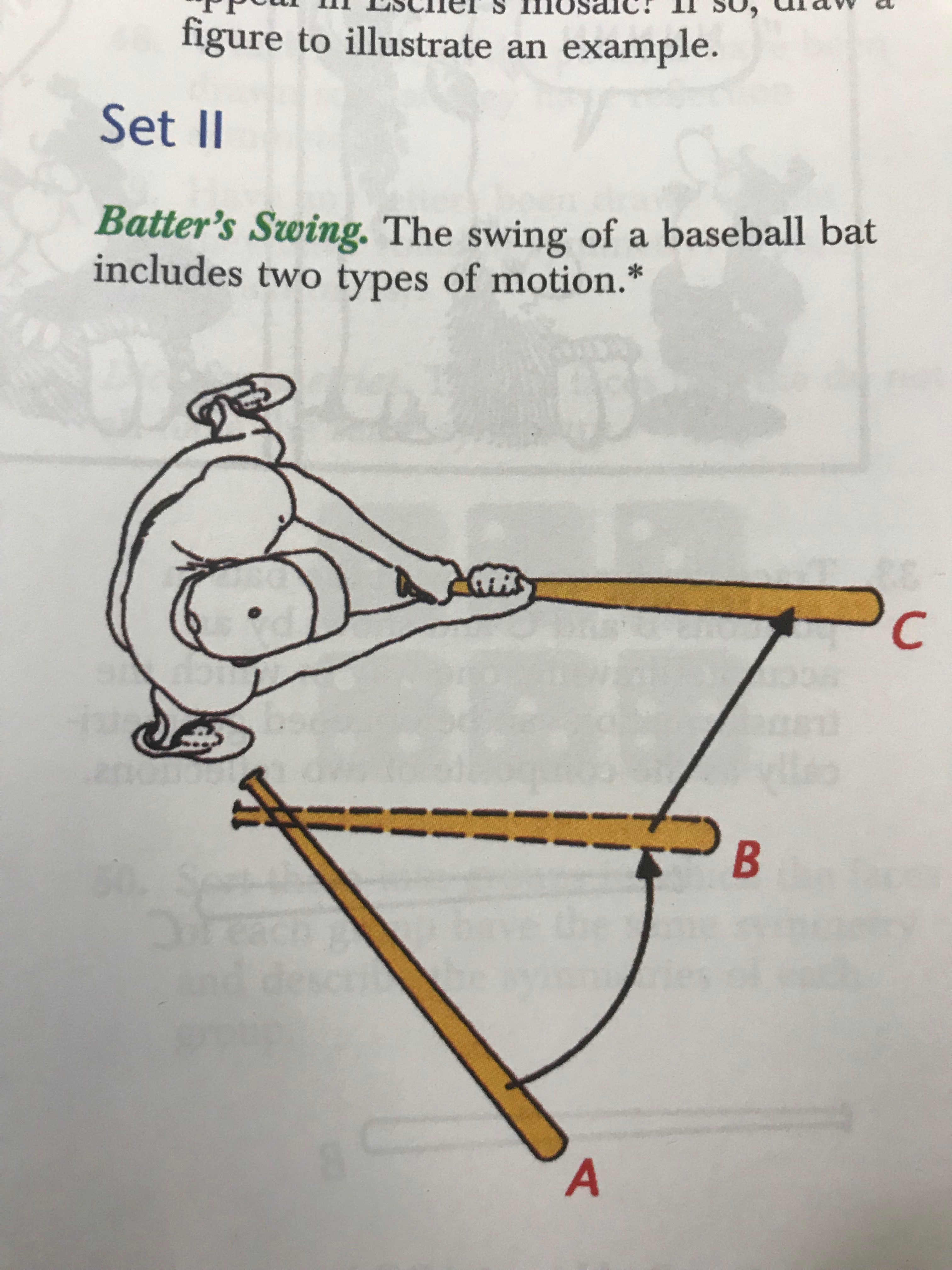Question from Jennifer:
Chap 8 review
#32
We aren’t sure how the answer would be 24mm.
This is not explained well in the textbook.
First – the translation is the movement of the bat from position B to position C (see chap 8, Lesson 2) A translation is two rotations around a parallel line – or in other words, we moved it over from one place to another.
So he is asking, how far did it move. Or, what is the length of the black line moving from B to C.
Since we can measure the bat to be about 42 mm (with a ruler), the length of the black line is about 24 mm using the same ruler.

Posted on

## Geometry Ch5.1 #29

Question from Courtney

I’m having trouble with Chapter 5, Lesson 1, Problem 29.
I have worked the problem, I know what the answer should be, but given the figure, I don’t understand how that could be false.
Without the figure, I understand it.

You can never assume anything. You only know what you are given in the definition. Don’t trust the figure. If it doesn’t tell you, you don’t know it. So for problem # 29 we know the following:

It’s a line
AB is less than BC
BC is less than CD
CD is less than DE

While the lengths in the figure look similar (or even equal), we don’t know that they really are similar or equal. Don’t trust anything but the defined statements in the problem and marked items in a figure.

Here is the question to think about. Given the definitions AB < BC < …. can you draw the figure such that the last DE is much larger.

Put some numbers in it and think inches.

AB = 1
BC = 2
CD = 3
DE = 500

Does that fit the definition of the problem? (Not the figure – the definition?)

Again – figures are often only ONE example that fits the problem – but they do not show every example.

Posted on

## Geometry Textbook Evaluations

Look for the following material or heading in the contents.

• Reasoning and Proof
· Proofs
· Deductive reasoning
· Direct and indirect proofs
• Lines
· Parallel and Perpendicular Lines
· Angles
• Triangles
· Congruent
· Isosceles
· Equilateral
· ASA and SAS
· Parallelograms
· Rectangles
· Squares
· Trapezoids
• Area
· Squares
· Rectangles
· Triangles
• Similarity
· Ratio and proportion
· Similar figures
• Right Triangle Trigonometry
· Pythagorean Theorem
· Proportions
· Tangent, Sine, and Cosine
• Surface Area and Volume
· Geometric solids
· Rectangular solids
· Spheres
• Circles